# RD Sharma Solutions for Class 9 Maths Chapter 14 Quadrilaterals Exercise 14.4

RD Sharma class 9 Mathematics chapter 14 exercise 14.4 solutions for Quadrilaterals are provided here. In this exercise, students will learn about useful facts about triangle and theorems results of triangle.

Some important results:

1. The line segment joining the mid-points of any two sides of a triangle is parallel to the third side and equal to half of it.

2. The line drawn through the mid-point of one side of a triangle, parallel to another side, intersects the 3rd side at its mid-point.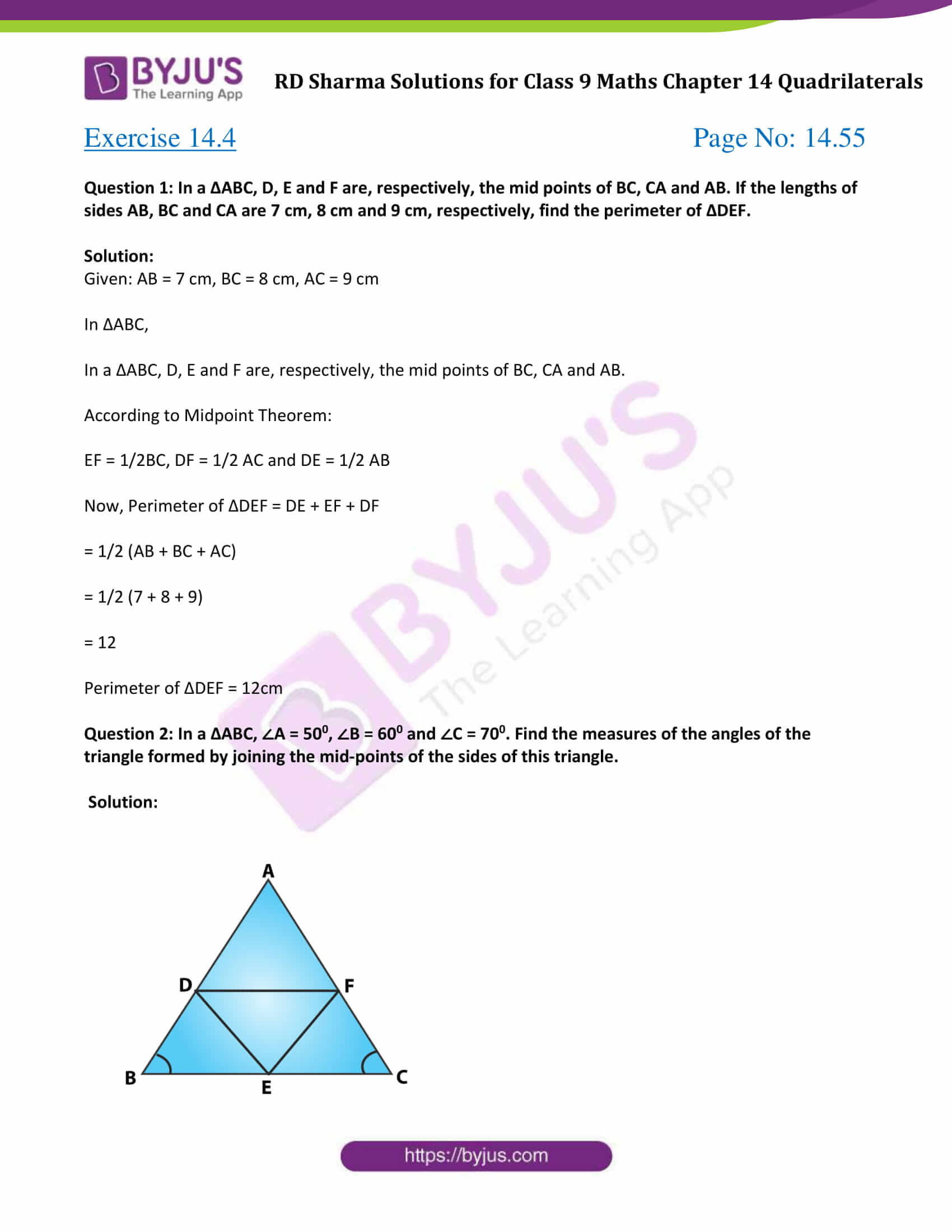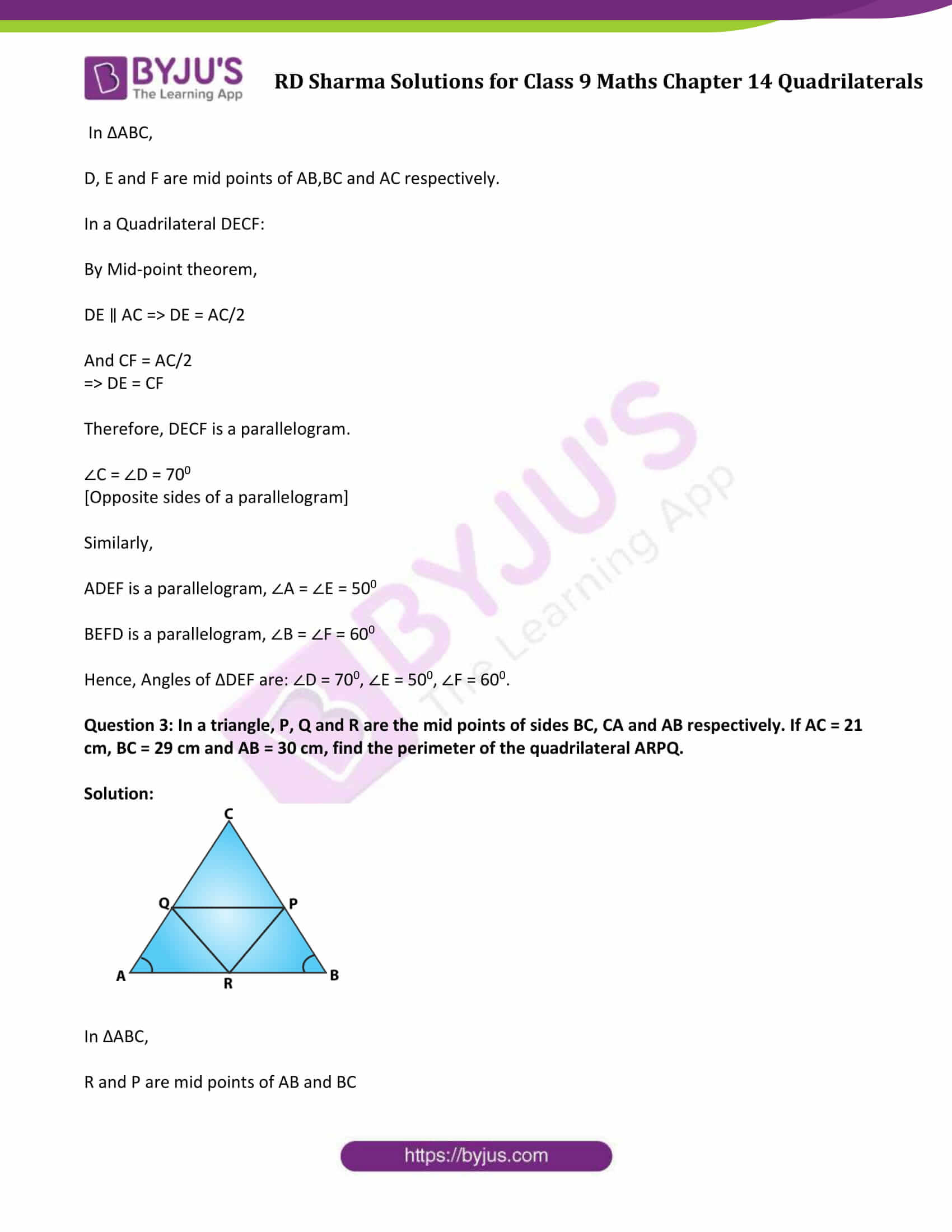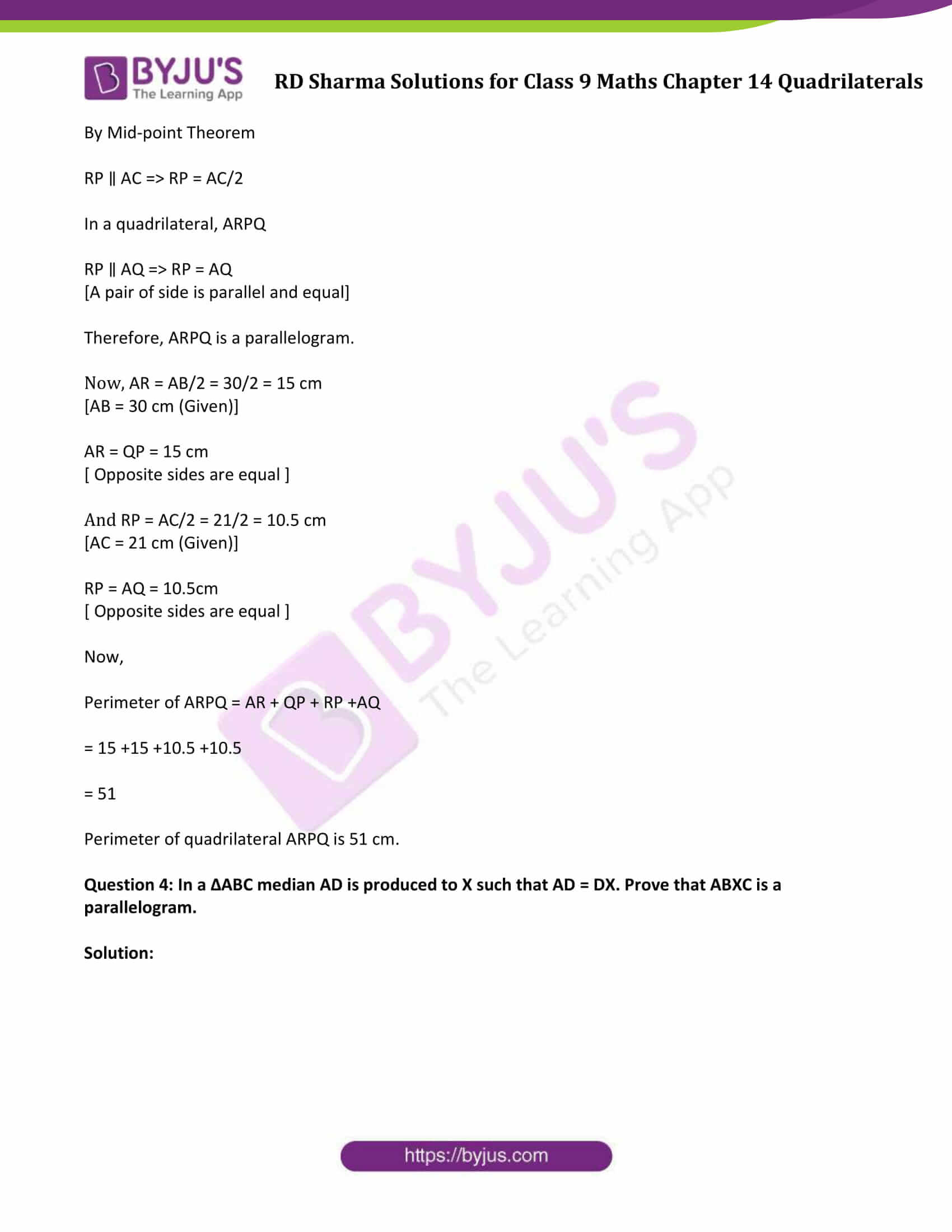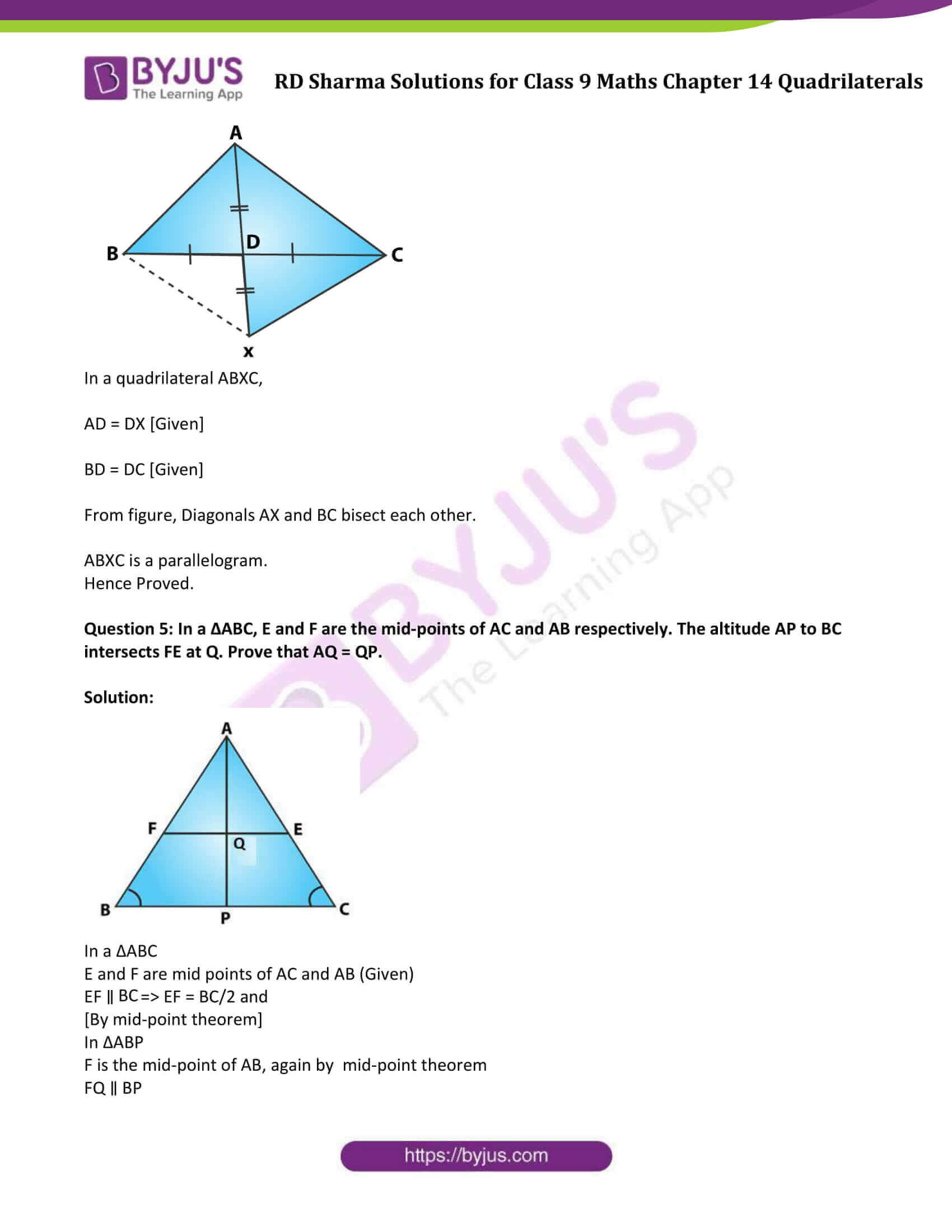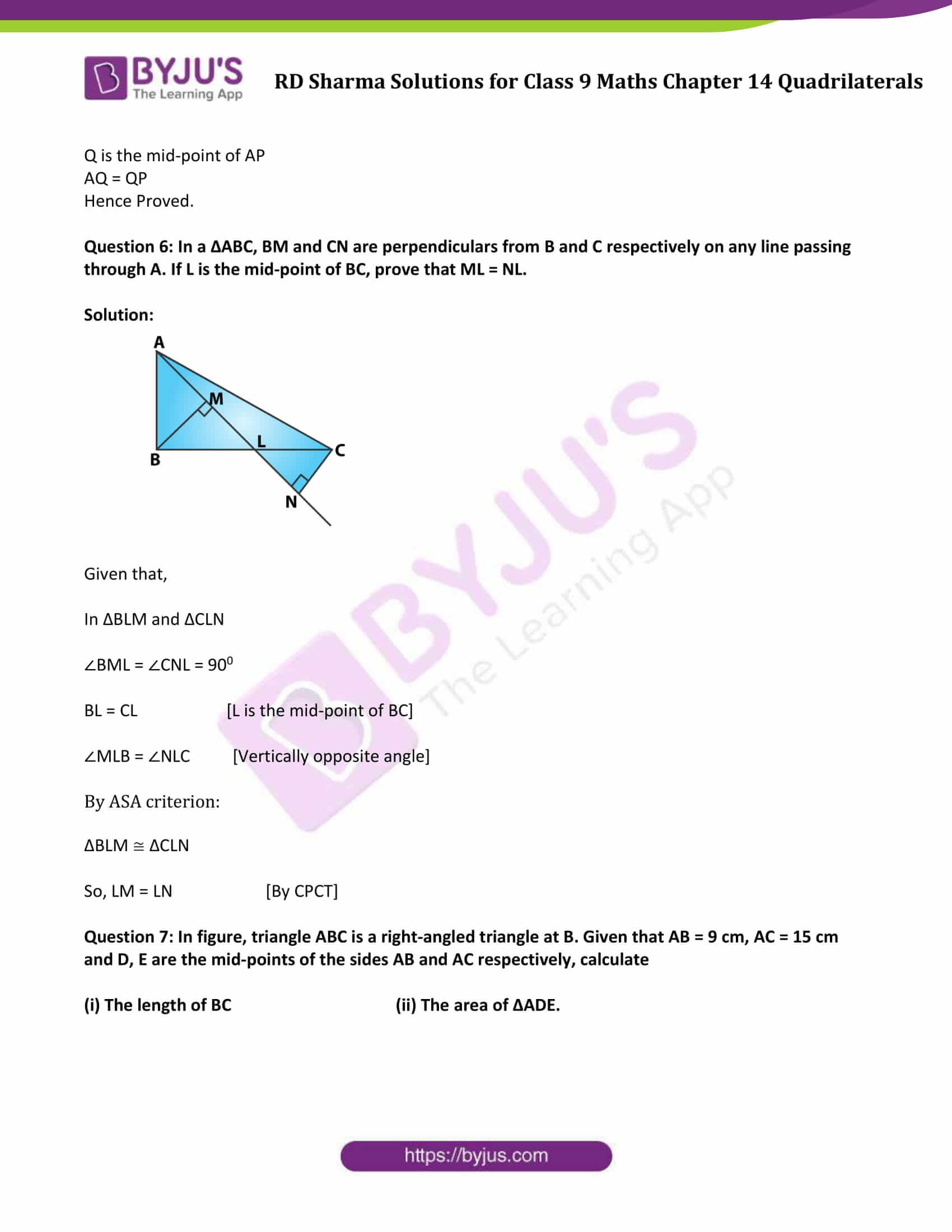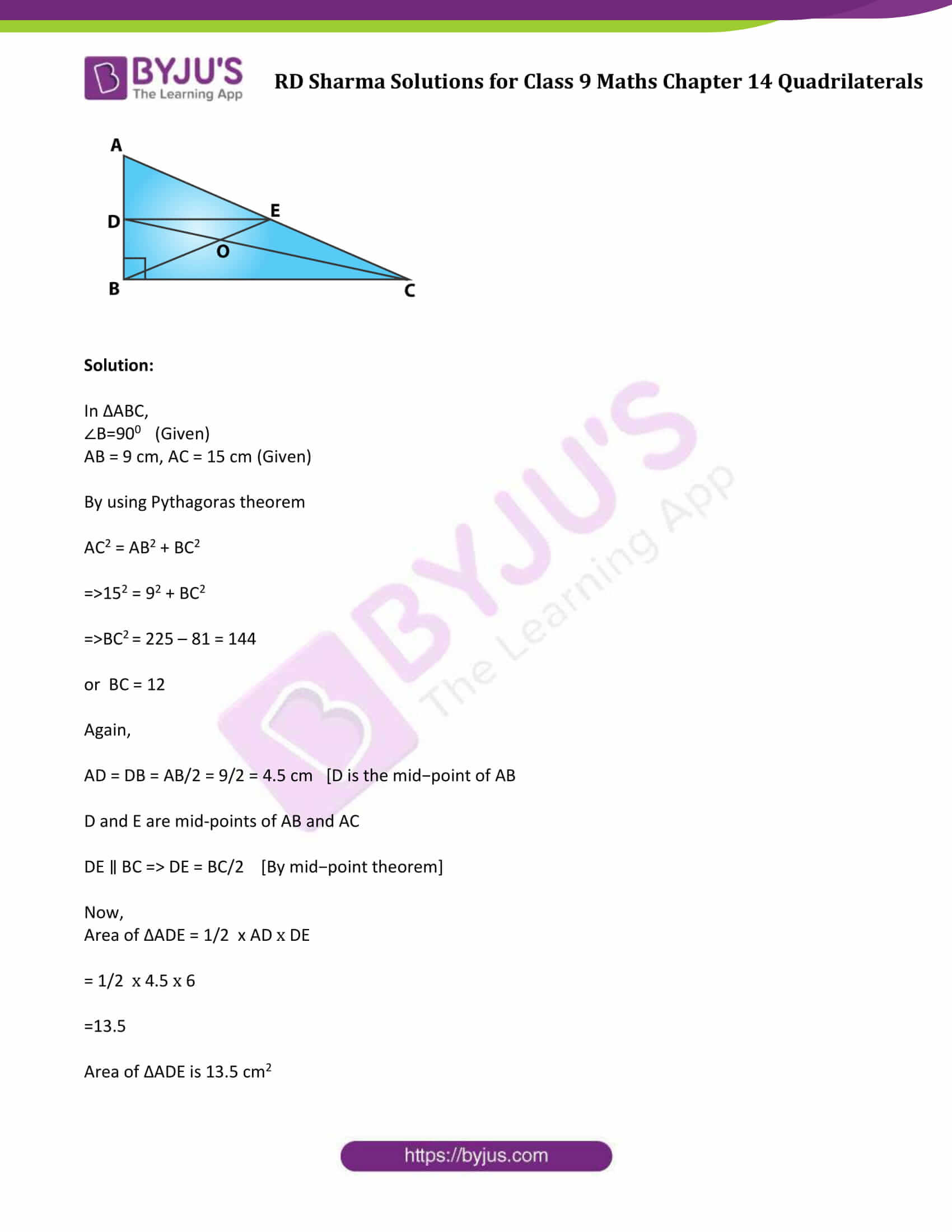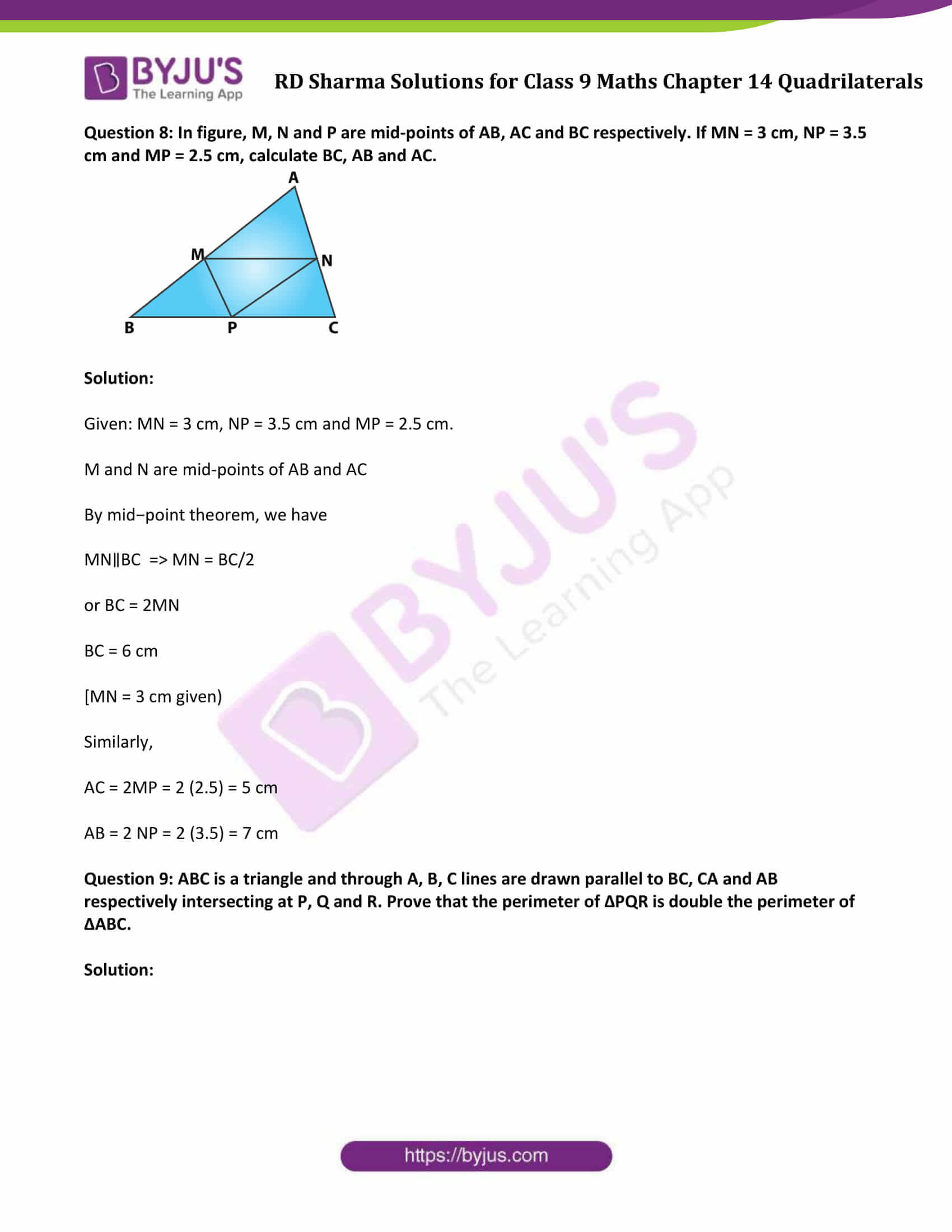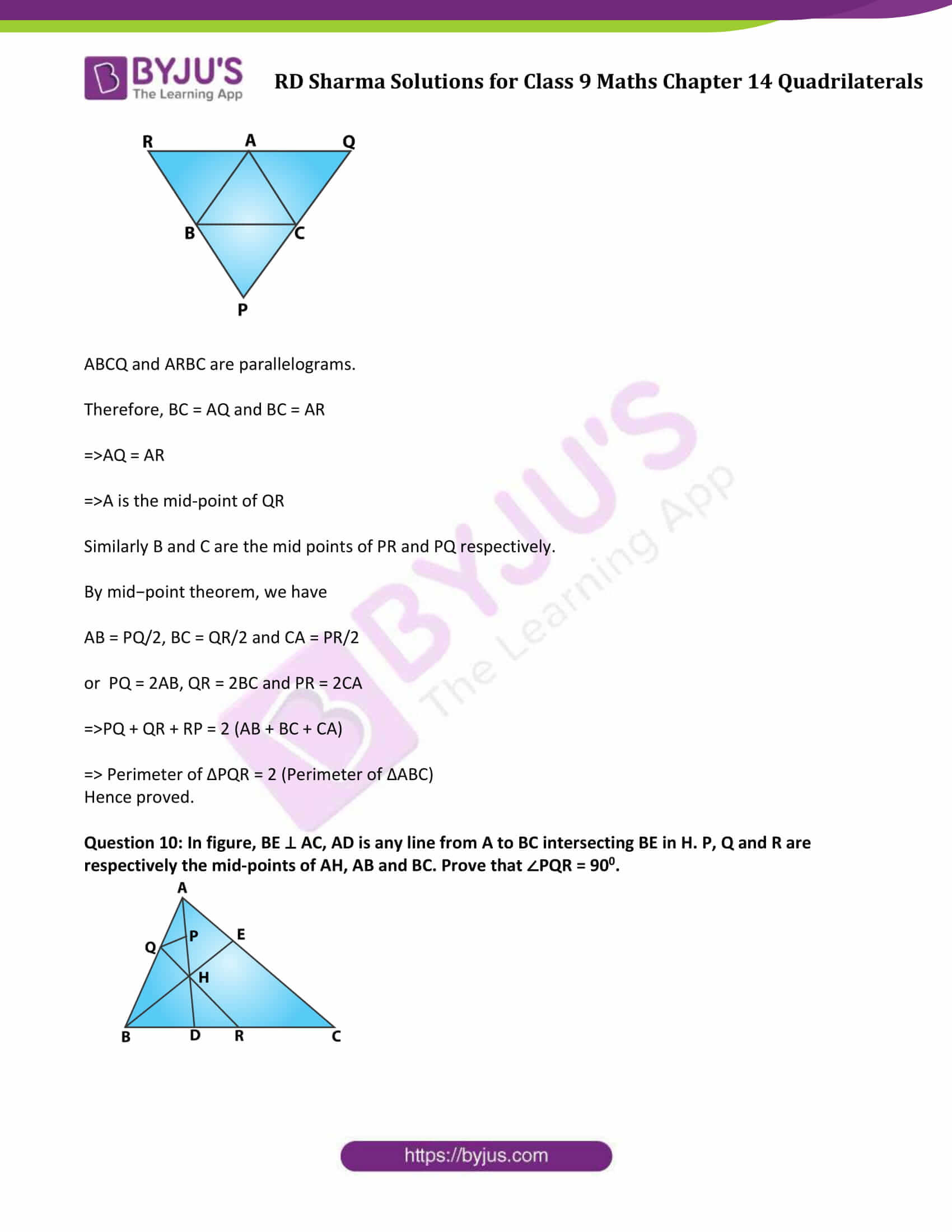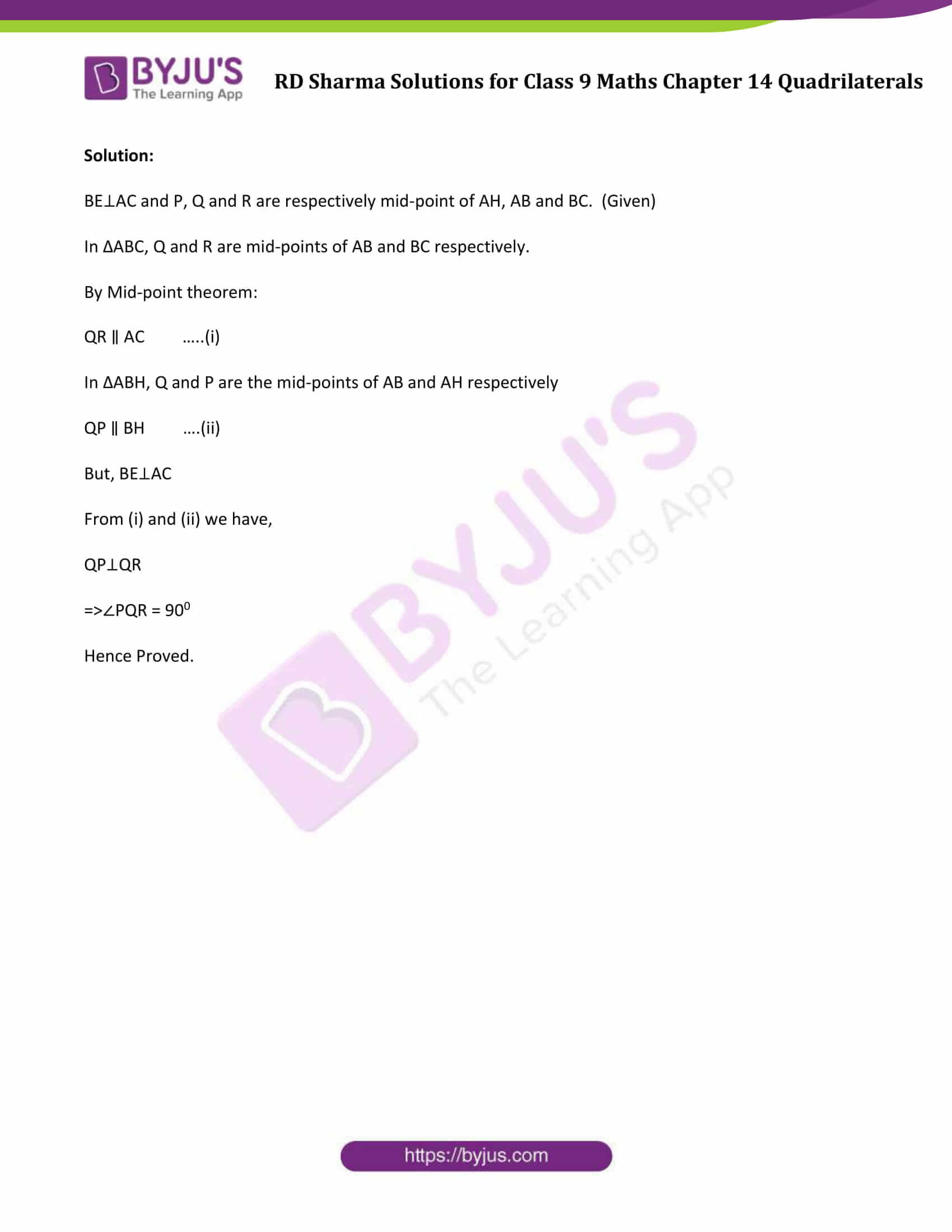### Access Answers to Maths RD Sharma Solutions for Class 9 Chapter 14 Quadrilaterals Exercise 14.4 Page number 14.55

Question 1: In a ΔABC, D, E and F are, respectively, the mid points of BC, CA and AB. If the lengths of sides AB, BC and CA are 7 cm, 8 cm and 9 cm, respectively, find the perimeter of ΔDEF.

Solution:

Given: AB = 7 cm, BC = 8 cm, AC = 9 cm

In ∆ABC,

In a ΔABC, D, E and F are, respectively, the mid points of BC, CA and AB.

According to Midpoint Theorem:

EF = 1/2BC, DF = 1/2 AC and DE = 1/2 AB

Now, Perimeter of ∆DEF = DE + EF + DF

= 1/2 (AB + BC + AC)

= 1/2 (7 + 8 + 9)

= 12

Perimeter of ΔDEF = 12cm

Question 2: In a ΔABC, ∠A = 500, ∠B = 600 and ∠C = 700. Find the measures of the angles of the triangle formed by joining the mid-points of the sides of this triangle.

Solution: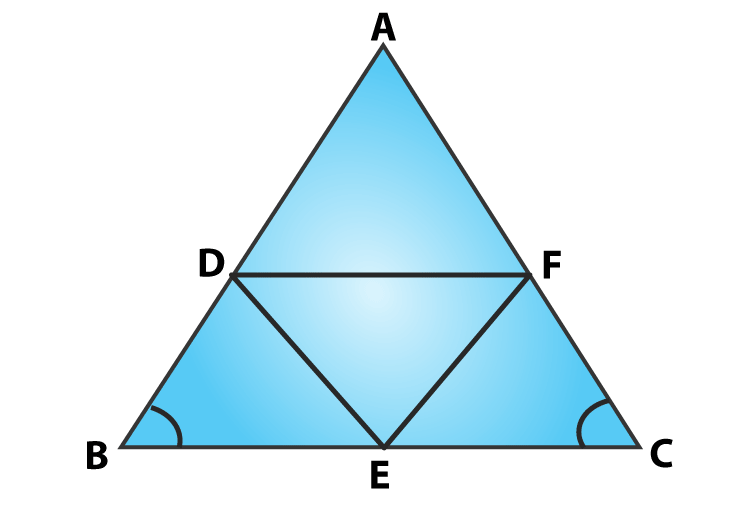In ΔABC,

D, E and F are mid points of AB,BC and AC respectively.

By Mid-point theorem,

DE ∥ AC ⇒ DE = AC/2

And CF = AC/2

⇒ DE = CF

Therefore, DECF is a parallelogram.

∠C = ∠D = 700

[Opposite sides of a parallelogram]

Similarly,

ADEF is a parallelogram, ∠A = ∠E = 500

BEFD is a parallelogram, ∠B = ∠F = 600

Hence, Angles of ΔDEF are: ∠D = 700, ∠E = 500, ∠F = 600.

Question 3: In a triangle, P, Q and R are the mid points of sides BC, CA and AB respectively. If AC = 21 cm, BC = 29 cm and AB = 30 cm, find the perimeter of the quadrilateral ARPQ.

Solution: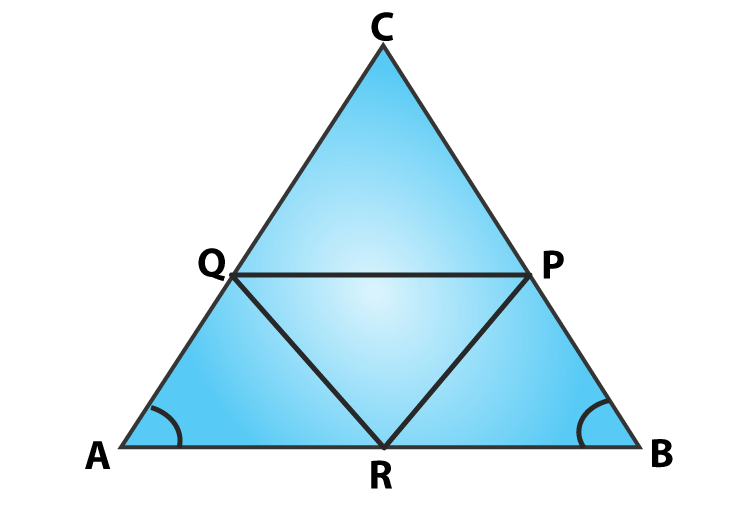In ΔABC,

R and P are mid points of AB and BC

By Mid-point Theorem

RP ∥ AC ⇒ RP = AC/2

RP ∥ AQ ⇒ RP = AQ

[A pair of side is parallel and equal]

Therefore, ARPQ is a parallelogram.

Now, AR = AB/2 = 30/2 = 15 cm

[AB = 30 cm (Given)]

AR = QP = 15 cm

[ Opposite sides are equal ]

And RP = AC/2 = 21/2 = 10.5 cm

[AC = 21 cm (Given)]

RP = AQ = 10.5cm

[ Opposite sides are equal ]

Now,

Perimeter of ARPQ = AR + QP + RP +AQ

= 15 +15 +10.5 +10.5

= 51

Perimeter of quadrilateral ARPQ is 51 cm.

Question 4: In a ΔABC median AD is produced to X such that AD = DX. Prove that ABXC is a parallelogram.

Solution: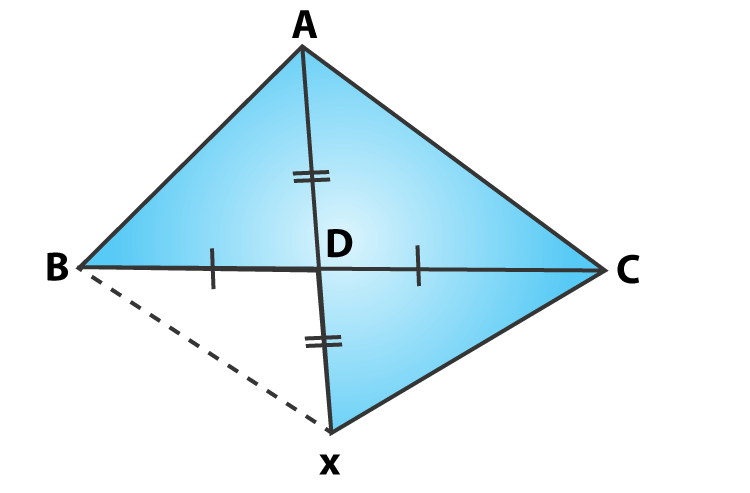BD = DC [Given]

From figure, Diagonals AX and BC bisect each other.

ABXC is a parallelogram.

Hence Proved.

Question 5: In a ΔABC, E and F are the mid-points of AC and AB respectively. The altitude AP to BC intersects FE at Q. Prove that AQ = QP.

Solution: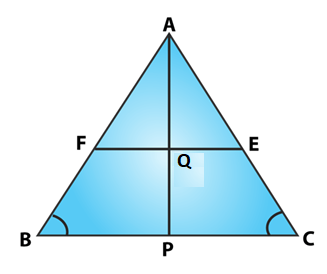In a ΔABC

E and F are mid points of AC and AB (Given)

EF ∥ BC ⇒ EF = BC/2 and

[By mid-point theorem]

In ΔABP

F is the mid-point of AB, again by mid-point theorem

FQ ∥ BP

Q is the mid-point of AP

AQ = QP

Hence Proved.

Question 6: In a ΔABC, BM and CN are perpendiculars from B and C respectively on any line passing through A. If L is the mid-point of BC, prove that ML = NL.

Solution: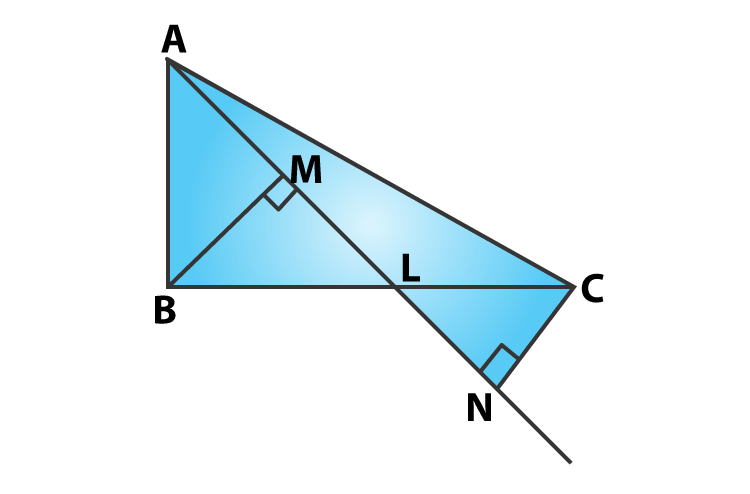Given that,

In ΔBLM and ΔCLN

∠BML = ∠CNL = 900

BL = CL [L is the mid-point of BC]

∠MLB = ∠NLC [Vertically opposite angle]

By ASA criterion:

ΔBLM ≅ ΔCLN

So, LM = LN [By CPCT]

Question 7: In figure, triangle ABC is a right-angled triangle at B. Given that AB = 9 cm, AC = 15 cm and D, E are the mid-points of the sides AB and AC respectively, calculate

(i) The length of BC (ii) The area of ΔADE.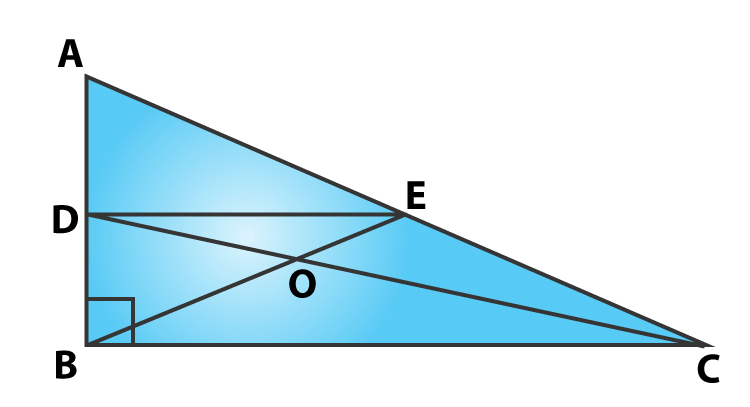Solution:

In ΔABC,

∠B=900 (Given)

AB = 9 cm, AC = 15 cm (Given)

By using Pythagoras theorem

AC2 = AB2 + BC2

⇒152 = 92 + BC2

⇒BC2 = 225 – 81 = 144

or BC = 12

Again,

AD = DB = AB/2 = 9/2 = 4.5 cm [D is the mid−point of AB

D and E are mid-points of AB and AC

DE ∥ BC ⇒ DE = BC/2 [By mid−point theorem]

Now,

= 1/2 x 4.5 x 6

=13.5

Area of ΔADE is 13.5 cm2

Question 8: In figure, M, N and P are mid-points of AB, AC and BC respectively. If MN = 3 cm, NP = 3.5 cm and MP = 2.5 cm, calculate BC, AB and AC.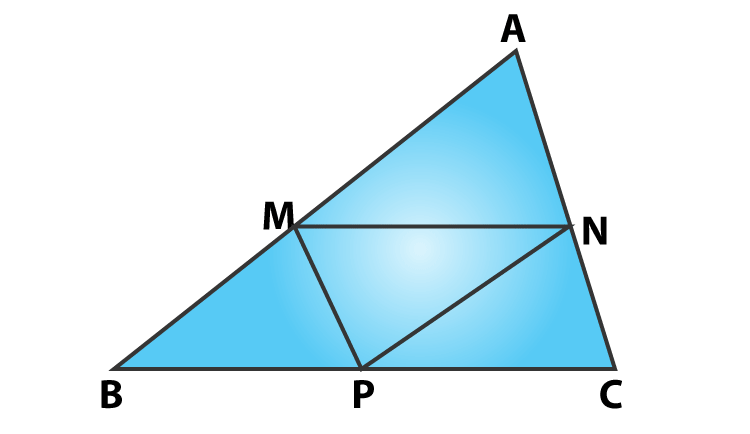Solution:

Given: MN = 3 cm, NP = 3.5 cm and MP = 2.5 cm.

M and N are mid-points of AB and AC

By mid−point theorem, we have

MN∥BC ⇒ MN = BC/2

or BC = 2MN

BC = 6 cm

[MN = 3 cm given)

Similarly,

AC = 2MP = 2 (2.5) = 5 cm

AB = 2 NP = 2 (3.5) = 7 cm

Question 9: ABC is a triangle and through A, B, C lines are drawn parallel to BC, CA and AB respectively intersecting at P, Q and R. Prove that the perimeter of ΔPQR is double the perimeter of ΔABC.

Solution: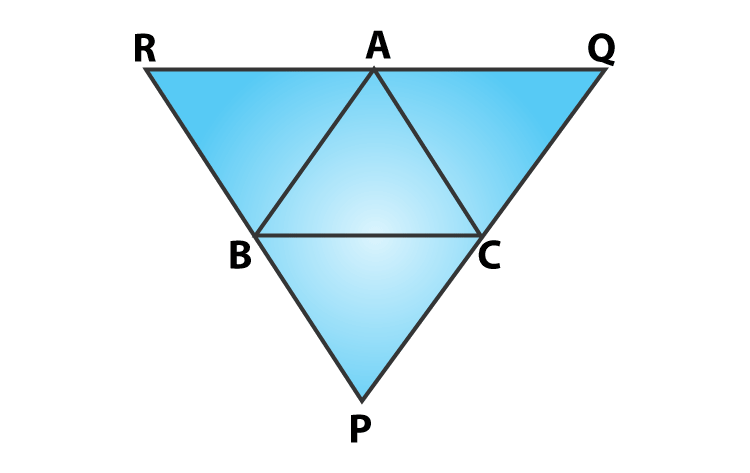ABCQ and ARBC are parallelograms.

Therefore, BC = AQ and BC = AR

⇒AQ = AR

⇒A is the mid-point of QR

Similarly B and C are the mid points of PR and PQ respectively.

By mid−point theorem, we have

AB = PQ/2, BC = QR/2 and CA = PR/2

or PQ = 2AB, QR = 2BC and PR = 2CA

⇒PQ + QR + RP = 2 (AB + BC + CA)

⇒ Perimeter of ΔPQR = 2 (Perimeter of ΔABC)

Hence proved.

Question 10: In figure, BE ⊥ AC, AD is any line from A to BC intersecting BE in H. P, Q and R are respectively the mid-points of AH, AB and BC. Prove that ∠PQR = 900.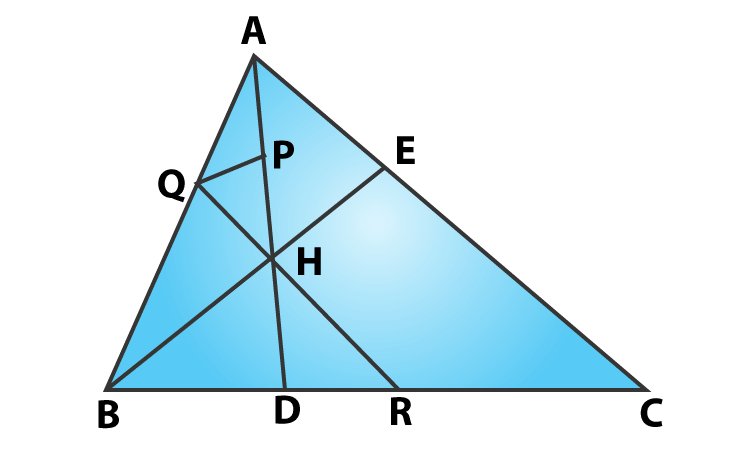Solution:

BE⊥AC and P, Q and R are respectively mid-point of AH, AB and BC. (Given)

In ΔABC, Q and R are mid-points of AB and BC respectively.

By Mid-point theorem:

QR ∥ AC …..(i)

In ΔABH, Q and P are the mid-points of AB and AH respectively

QP ∥ BH ….(ii)

But, BE⊥AC

From (i) and (ii) we have,

QP⊥QR

⇒∠PQR = 900

Hence Proved.

## Access other exercise solutions of Class 9 Maths Chapter 14 Quadrilaterals

Exercise 14.1 Solutions

Exercise 14.2 Solutions

Exercise 14.3 Solutions

Exercise VSAQs Solutions

## RD Sharma Solutions for Class 9 Maths Chapter 14 Quadrilaterals Exercise 14.4

Class 9 Maths Chapter 14 Quadrilaterals Exercise 14.4 is based on the following topics: